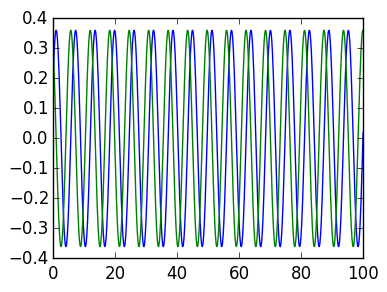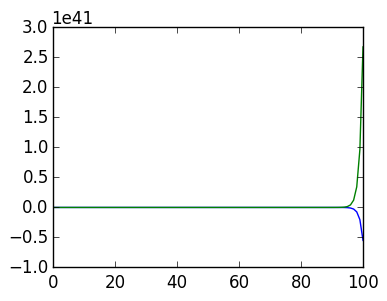# Lecture 23: The SVD and Condition Numbers, The Matrix Exponential¶

In this lecture we discuss more on the SVD, and also discuss the role of eigenvalue decompositions.

## The SVD and condition numbers¶

Recall for $A \in \mathbb R^{n \times n}$ we have

$$\|A\|_2 = \sigma_1,$$

that is the norm is the largest singular value. On the other hand, we have

$$A^{-1} = (U \Sigma V^\top)^{-1} = V \Sigma^{-1} U^\top = V \begin{pmatrix} \sigma_1^{-1} \cr & \ddots \cr && \sigma_n^{-1} \end{pmatrix} U^\top,$$

hence

$$\|A^{-1}\|_2 = \sigma_n^{-1},$$

i.e., one over the smallest singular value. Thus we have the condition number being the ratio of the largest to the smallest singular value:

$$\kappa(A) \triangleq \|A\|_2 \|A^{-1}\|_2 = {\sigma_1 \over \sigma_n}$$

#### Hilbert matrix example, revisited¶

We saw before that the Hilbert matrix was very badly conditioned. We can confirm that the condition number is the ratio of largest to smallest singular value:

In :
n=10

H=zeros(n,n)
for k=1:(2n-1)
for j=1:k
if (k-j+1)≤n && (j ≤ n)
H[k-j+1,j]=1/k
end
end
end

σ=svdvals(H)

cond(H),σ/σ[end]

Out:
(1.602475208417804e13,1.602475208417804e13)

The condition number tells us the worst case relative error in applying H, But this is an overestimate for generic vectors:

In :
x=rand(n)

Δx=0.00001*rand(n)

norm(H*(x+Δx)-H*x)/norm(H*x), cond(H)*norm(Δx)/norm(x)

Out:
(5.633202480002264e-6,9.192597301117952e7)

But we can use the SVD to find the vector that is highly sensitive to perturbation. The key observation is the the $k$th column of $V$ is magnified by $\sigma_k$. This means that the last column is scaled by the smallest singular value:

In :
U,σ,V=svd(H)
Σ=diagm(σ)
norm(H*V[:,end]),norm(σ[end])

Out:
(1.0932569988896404e-13,1.0932585172377964e-13)

Any small perturbation of this vector ignites other singular values, including the largest singular value. Thus the norm of the perturbation is substantially different than the unperturbed vector:

In :
x=V[:,end]
Δx=0.00001*rand(n)
norm(H*(x+Δx))

Out:
1.904075675613028e-5

The relative error of this perturbation is now very large, as reflected by the condition number:

In :
x=V[:,end]
Δx=0.00001*rand(n)
norm(H*(x+Δx)-H*x)/norm(H*x), cond(H)*norm(Δx)/norm(x)

Out:
(2.425330000204698e8,3.0140514377512914e8)

## Eigenvalues and matrix powers¶

We now turn our attention to eigenvalue decompositions. Assume that our matrix $A \in \mathbb R^{n \times n}$ has an eigenvalue decomposition

$$A = V \Lambda V^{-1}$$

where $\Lambda$ is diagonal.

An important property of the eigenvalue decomposition is that matrix powers are diagonalized:

$$A^s = (V \Lambda V^{-1})^s = V \Lambda V^{-1} V \Lambda V^{-1} \cdots V \Lambda V^{-1} = V \Lambda^s V^{-1} = V\begin{pmatrix} \lambda_1^s \cr & \ddots \cr && \lambda_n^s \end{pmatrix}V^{-1}$$

Numerically, we want to restrict our attention to the case where $V = Q$ is orthogonal:

$$A = Q \Lambda Q^\top$$

as otherwise the condition number of $V$ may introduce large errors.

### Time evolution of constant coefficient linear ODEs¶

Consider the initial-value ODE

$$\mathbf u'(t) = A \mathbf u(t), \qquad \mathbf u(0) = \mathbf u_0$$

where $\mathbf u_0 \in \mathbb R^n$ is an initial condition. In the scalar case, this is

$$u'(t) = a u(t), \qquad u(0) = u_0$$

which is solved via the exponential $e^{a t} u_0$. The key defining property is that

$$e^x = \sum_{k=0}^\infty {x^k \over k!}$$

This translates naturally to the matrix exponential, defined via matrix powers:

$$e^A \triangleq \sum_{k=0}^\infty {A^k \over k!}$$

Exercise (Advanced) Use the properties of norms of the matrix $A$ to prove that this sum converges and that the following manipulations are mathematically justified.

We thus arrive at the solution to our ODE:

$$\mathbf u'(t) = e^{\mathbf A t} \mathbf u_0$$

Indeed, we have

$$\mathbf u(0) = e^{\mathbf A 0} \mathbf u_0 = \mathbf u_0$$

and

$$\mathbf u'(t) = {d \over dt}[e^{\mathbf A t} \mathbf u_0] = \sum_{k=1}^\infty {Ak(At)^{k-1} \over k!}\mathbf u_0 = A \sum_{k=1}^\infty {(At)^{k-1} \over (k-1)!}\mathbf u_0 = Ae^{\mathbf A t} \mathbf u_0 = A \mathbf u(t).$$

The matrix exponential is expm in Julia. We can use this to plot solve ODEs:

In :
A=[0 1;
-1 0]

## u' = Au,  u(0) = [.2,.3]

u0=[.2,.3]
t=100.
expm(A*t)*u0   #expm is the matrix exponential

Out:
2-element Array{Float64,1}:
0.0205541
0.359969 

Here is a plot of an oscillatory solution:

In :
using PyPlot

T=linspace(0.,100.,1000)
plot(T,Float64[(expm(A*t)*u0) for t in T])
plot(T,Float64[(expm(A*t)*u0) for t in T])Out:
1-element Array{Any,1}:
PyObject <matplotlib.lines.Line2D object at 0x31743e310>

One simple approach to calculating expm is the Taylor series:

In :
function expm_taylor(A,t,u0,N)
ret=u0
v0=u0
for k=1:N
v0=A*t*v0/k
ret+=v0
end
ret
end

Out:
expm_taylor (generic function with 1 method)

Unfortunately, it quickly breaks down for large t:

In :
using PyPlot

T=linspace(0.,100.,100)
plot(T,Float64[expm_taylor(A,t,u0,100) for t in T])
plot(T,Float64[expm_taylor(A,t,u0,100) for t in T])Out:
1-element Array{Any,1}:
PyObject <matplotlib.lines.Line2D object at 0x3175094d0>

Instead, we will use the eigenvalue decomposition $A = V \Lambda V^{-1}$, so that

$$e^A = \sum_{k=0}^\infty {A^k \over k!} = V\sum_{k=0}^\infty {\Lambda^k \over k!} V^{-1} = V e^{\Lambda} V^{-1} = \begin{pmatrix} e^\lambda_1 \cr & \ddots \cr && e^\lambda_n \end{pmatrix} V^{-1}$$

In otherwords, if we can calculate a scalar exponential and an eigenvalue decomposition, we can calculate the matrix exponential. :

In :
λ,Q=eig(A)
Q*diagm([exp(λ*t),exp(λ*t)])*Q'*u0   - expm(A*t)*u0

Out:
2-element Array{Complex{Float64},1}:
-3.88578e-16+0.0im
4.44089e-16+0.0im